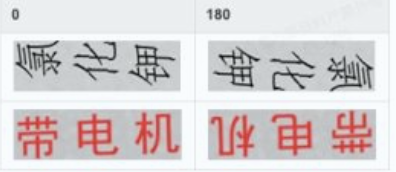## 关于提高OCR识别准确率的一些优化（二）

2021/6/26 9:47:42 人评论

### 关于提高OCR识别准确率的一些优化（二）

• 前言
• 一、为什么要判断方向？
• 1.方法介绍
• 2、实际效果测试
• 3、文本框检测实际效果
• 4、结果分析
• 5、解决办法
• 6、方向检测测试结果
• 1.训练
• 2.测试结果分析
• 四、后续优化方向
• 总结

# 一、为什么要判断方向？

## 1.方法介绍

0和180度数据样本例子## 2、实际效果测试## 3、文本框检测实际效果## 4、结果分析

3、结合以上分析结果，我们可以利用矩形框的长宽比来确定文本的方向。

## 5、解决办法

``````def get_real_rotation(rect_list):
w_div_h_sum = 0
count = 0
for rect in rect_list:
p0 = rect	#p0,p1,p2,p3为矩形框的四个角点坐标
p1 = rect
p2 = rect
p3 = rect
width = abs(p1 - p0)
height = abs(p3 - p0)
w_div_h =  width / height	#计算长款比
if abs(w_div_h - 1.0) < 0.5:	#过滤长宽比差距接近1的矩形框，以免影响检测准确度
count +=1
continue
w_div_h_sum += w_div_h
if w_div_h_sum / (len(rect_list) - count) >= 1.5:	#长宽比大于1.5的，则paddleocr的检测结果是可信的
return 1
else:
return 0

def get_img_real_angle(img_path):
angle_cls = ocr.ocr(img_path, det=False, rec=False, cls=True)	#得到paddleocr检测角度
print(angle_cls)
rect_list = ocr.ocr(img_path, rec=False)	#得到所有矩形框的角点坐标
real_angle_flag = get_real_rotation_new(rect_list)

if angle_cls == '0':
if real_angle_flag:
ret_angle = 0
else:
ret_angle = 270
if angle_cls == '180':
if real_angle_flag:
ret_angle = 180
else:
ret_angle = 90
return ret_angle

``````

## 6、方向检测测试结果

1、跑了几十张图片测试了一下，发现只有60%的准确率，效果不怎么好。
2、可能的原因：

## 1.训练

2、于是我马上拿官方例子试了一下，代码如下：

``````#%%
# 设置使用0号GPU卡（如无GPU，执行此代码后仍然会使用CPU训练模型）
import matplotlib
matplotlib.use('Agg')
import os
os.environ['CUDA_VISIBLE_DEVICES'] = '0'
# %%
train_transforms = transforms.Compose([
transforms.RandomCrop(crop_size=224),
transforms.RandomHorizontalFlip(),
transforms.Normalize()
])
eval_transforms = transforms.Compose([
transforms.ResizeByShort(short_size=256),
transforms.CenterCrop(crop_size=224),
transforms.Normalize()
])
# %%
train_dataset = pdx.datasets.ImageNet(
transforms=train_transforms,
shuffle=True)
eval_dataset = pdx.datasets.ImageNet(
shuffle=True,
transforms=eval_transforms)
# %%
num_classes = len(train_dataset.labels)
model = pdx.cls.MobileNetV3_small_ssld(num_classes=num_classes)

#%%
model.train(num_epochs=20,
train_dataset=train_dataset,
train_batch_size=32,
eval_dataset=eval_dataset,
lr_decay_epochs=[4, 6, 8],
save_dir='output/mobilenetv3_small_ssld',
use_vdl=True)
# %%
print("Predict Result: ", result)

``````

3、运行之后。。。。。。。。。。。。。。。。。5、经过一番排查，发现路径完全是对的。我的机器是mac，所以我想有没有可能是系统的原因，于是我把代码拿到linux运行。

## 2.测试结果分析

1、每个类别使用200条数据训练，训练集准确率为24%
2、每个类别使用2000条数据训练，训练集准确率为40%
3、测试集准确率为10%

# 四、后续优化方向

1、经过一周的各种优化测试，都没有对ocr识别准确率有大的提升，但目前可以确定的是，转正后的图片识别效果是远高于未转正图片的，因此，后期还是会从方向去突破。